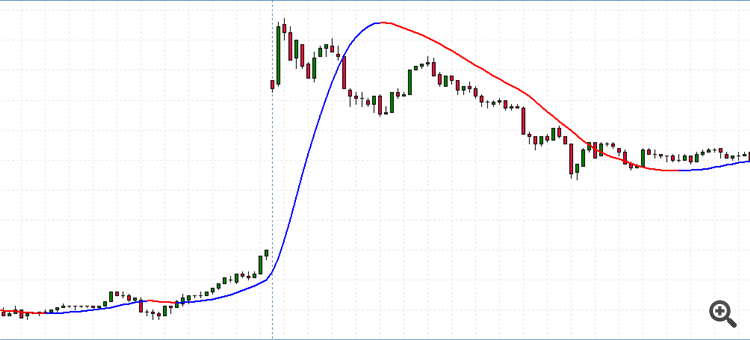"Reset" Moving Average daily (at Market open)52

Hello ,

Is there a way i could recode moving average indicators so that they reset every day? I often get wrong signals because of the market opening much higher/lower than the previous close.

Heres what i mean:

instead of this:   ( this example is using a HMA , but im interested in this for MA's in general)id like it to look something like this, similar to how a VWAP restarts every day.

Any tipps would be appreciated.

Thanks!19837

dodo3441: Is there a way i could recode moving average indicators so that they reset every day?
Yes you have to code it. Where computeAverage only processes that many bars, e.g. SMA(1) the close, SMA(2), ... SMA(extLength):

Just typed, not compiled not tested.

//+------------------------------------------------------------------+
//{ Generic / Utility functions                                      |
//+------------------------------------------------------------------+
//{ Typedefs
#define  INDEX    uint           // Zero based.
#define  COUNT    uint           //  One based.
#define  SECONDS  uint
//} Typedefs
#define  HR2400 (PERIOD_D1 * 60) // 86400 = 24 * 3600 = 1440 * 60
SECONDS     time(datetime when=0){        if(when == 0) when = TimeCurrent();
return SECONDS(when % HR2400);
}
datetime    date(datetime when=0){        if(when == 0) when = TimeCurrent();
return datetime(when - time(when) );
}
//+------------------------------------------------------------------+
//} Generic code                                                     |
//{ Specific code                                                    |
//+------------------------------------------------------------------+
int OnCalculate(...){
// Lookbacks
#define  LAST              0
#define  REDRAW_BAR_LAST   (LAST == 0)       // Redraw bar last.
#define  LOOKBACK          0                 // No LB before today.
int iBar = rates_total - MathMax(LOOKBACK, prev_calculated);

static datetime dToday=0;
static COUNT    nToday=0;
if(prev_calculated==0){ dToday=0;   }
while(iBar > LAST){ --iBar;
datetime prevDate=dToday; dToday=date(Time[iBar]);
if(dToday != prevDate) nToday=Bars-iBar;
INDEX    iToday=Bars - nToday;
COUNT    length=(int)iToday - iBar + 1;
computeAverage( buffer, iBar, MathMin(extLength, length) );
}
return rates_total-iBar-REDRAW_BAR_LAST;
}  // OnCalculate
MT4 code, adjust Time/Bars for MT5.
See How to do your lookbacks correctly.